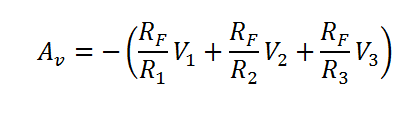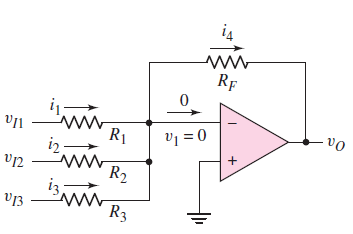# Output of a Summing Op-Amp calculator

This CalcTown calculator calculates the output of an ideal summing op-amp amplifier.

K-Ohm
K-Ohm
K-Ohm
K-Ohm
V
V
V

#### Result

V